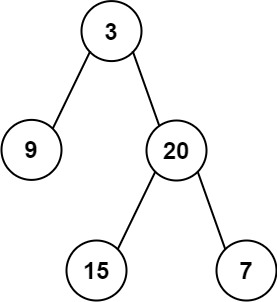# 404.sum-of-left-leaves

## Statement

• Difficulty: Easy
• Tag: `树` `深度优先搜索` `广度优先搜索` `二叉树```````输入: root = [3,9,20,null,null,15,7]

``````

``````输入: root = 

``````

• 节点数在 `[1, 1000]` 范围内
• `-1000 <= Node.val <= 1000`

• Link: Sum of Left Leaves
• Difficulty: Easy
• Tag: `Tree` `Depth-First Search` `Breadth-First Search` `Binary Tree`

Given the `root` of a binary tree, return the sum of all left leaves.

A leaf is a node with no children. A left leaf is a leaf that is the left child of another node.

Example 1:``````Input: root = [3,9,20,null,null,15,7]
Output: 24
Explanation: There are two left leaves in the binary tree, with values 9 and 15 respectively.
``````

Example 2:

``````Input: root = 
Output: 0
``````

Constraints:

• The number of nodes in the tree is in the range `[1, 1000]`.
• `-1000 <= Node.val <= 1000`

## Solution

``````# Definition for a binary tree node.
# class TreeNode:
#     def __init__(self, val=0, left=None, right=None):
#         self.val = val
#         self.left = left
#         self.right = right
from typing import Optional

class Solution:
def __init__(self):
self.res = 0

def dfs(self, root: Optional[TreeNode], is_left: bool) -> None:
if not root:
return

son = 0
if root.left:
son += 1
self.dfs(root.left, True)

if root.right:
son += 1
self.dfs(root.right, False)

if son == 0 and is_left:
self.res += root.val

def sumOfLeftLeaves(self, root: Optional[TreeNode]) -> int:
self.dfs(root, False)
return self.res
``````